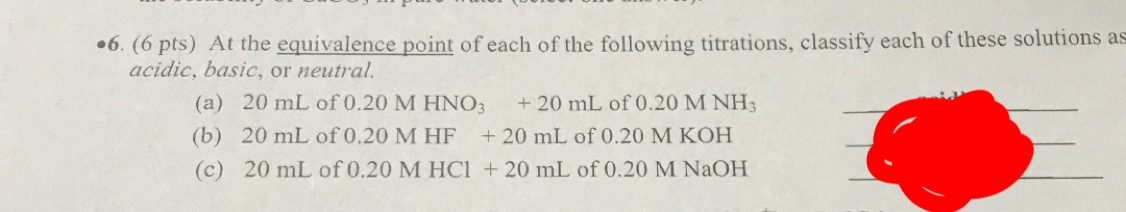# At the equivalence point of each of the following titrations, classify each of these solutions as acidic, basic or netural. (a) 20 mL of 0.20 M HNO3 + 20 mL of 0.20 M NH3 (b) 20 mL of 0.20 M HF + 20 mL of 0.20 M KOH (c) 20 mL of 0.20 M HCl + 20 mL of 0.20 M NaOH